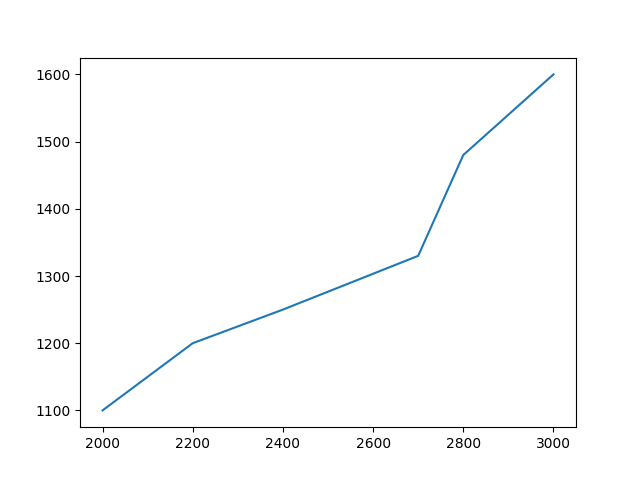## 31 Jan Matplotlib – Plotting

In this lesson, we will plot a line in Matplotlib. For the x and y input, let us consider a DataFrame. The following is the library to be imported for Pandas:

The following is the library to be imported for Matplotlib with the PyPlot sub module:

First, create a DataFrame. The Pandas DataFrame is a Two-dimensional, tabular data, table with rows and columns. The DataFrame() method is used for this purpose:

The columns of the DataFrame will be used for both the x and y coordinates of the plot():

Let us now see the complete example to plot in Matplotlib:

OutputIf you liked the tutorial, spread the word and share the link and our website Studyopedia with others.